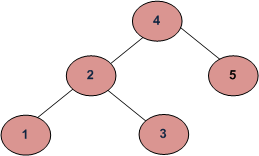Skip to content
Related Articles
Check if a Binary Tree is BST : Simple and Efficient Approach
• Difficulty Level : Easy
• Last Updated : 06 Nov, 2020

Given a Binary Tree, the task is to check whether the given binary tree is Binary Search Tree or not.
A binary search tree (BST) is a node-based binary tree data structure which has the following properties.

• The left subtree of a node contains only nodes with keys less than the node’s key.
• The right subtree of a node contains only nodes with keys greater than the node’s key.
• Both the left and right subtrees must also be binary search trees.

From the above properties it naturally follows that:

• Each node (item in the tree) has a distinct key.We have already discussed different approaches to solve this problem in the previous article.
In this article, we will discuss a simple yet efficient approach to solve the above problem.
The idea is to use Inorder traversal and keep track of the previously visited node’s value. Since the inorder traversal of a BST generates a sorted array as output, So, the previous element should always be less than or equals to the current element.
While doing In-Order traversal, we can keep track of previously visited Node’s value and if the value of the currently visited node is less than the previous value, then the tree is not BST.

Below is the implementation of the above approach:

## C++

 `// C++ program to check if a given tree is BST.``#include ``using` `namespace` `std;` `/* A binary tree node has data, pointer to``left child and a pointer to right child */``struct` `Node {``    ``int` `data;``    ``struct` `Node *left, *right;` `    ``Node(``int` `data)``    ``{``        ``this``->data = data;``        ``left = right = NULL;``    ``}``};` `// Utility function to check if Binary Tree is BST``bool` `isBSTUtil(``struct` `Node* root, ``int``& prev)``{``    ``// traverse the tree in inorder fashion and``    ``// keep track of prev node``    ``if` `(root) {``        ``if` `(!isBSTUtil(root->left, prev))``            ``return` `false``;` `        ``// Allows only distinct valued nodes``        ``if` `(root->data <= prev)``            ``return` `false``;` `        ``// Initialize prev to current``        ``prev = root->data;` `        ``return` `isBSTUtil(root->right, prev);``    ``}` `    ``return` `true``;``}` `// Function to check if Binary Tree is BST``bool` `isBST(Node* root)``{``    ``int` `prev = INT_MIN;``    ``return` `isBSTUtil(root, prev);``}` `/* Driver code*/``int` `main()``{``    ``struct` `Node* root = ``new` `Node(5);``    ``root->left = ``new` `Node(2);``    ``root->right = ``new` `Node(15);``    ``root->left->left = ``new` `Node(1);``    ``root->left->right = ``new` `Node(4);` `    ``if` `(isBST(root))``        ``cout << ``"Is BST"``;``    ``else``        ``cout << ``"Not a BST"``;` `    ``return` `0;``}`

## Java

 `// Java program to check if a given tree is BST.``class` `GFG {` `    ``static` `int` `prev = Integer.MIN_VALUE;``    ``/* A binary tree node has data, pointer to``    ``left child and a pointer to right child */``    ``static` `class` `Node {``        ``int` `data;``        ``Node left, right;` `        ``Node(``int` `data)``        ``{``            ``this``.data = data;``            ``left = right = ``null``;``        ``}``    ``};` `    ``// Utility function to check if Binary Tree is BST``    ``static` `boolean` `isBSTUtil(Node root)``    ``{``        ``// traverse the tree in inorder fashion and``        ``// keep track of prev node``        ``if` `(root != ``null``) {``            ``if` `(!isBSTUtil(root.left))``                ``return` `false``;` `            ``// Allows only distinct valued nodes``            ``if` `(root.data <= prev)``                ``return` `false``;` `            ``// Initialize prev to current``            ``prev = root.data;` `            ``return` `isBSTUtil(root.right);``        ``}` `        ``return` `true``;``    ``}` `    ``// Function to check if Binary Tree is BST``    ``static` `boolean` `isBST(Node root)``    ``{``        ``return` `isBSTUtil(root);``    ``}` `    ``/* Driver code*/``    ``public` `static` `void` `main(String[] args)``    ``{``        ``Node root = ``new` `Node(``5``);``        ``root.left = ``new` `Node(``2``);``        ``root.right = ``new` `Node(``15``);``        ``root.left.left = ``new` `Node(``1``);``        ``root.left.right = ``new` `Node(``4``);` `        ``if` `(isBST(root))``            ``System.out.print(``"Is BST"``);``        ``else``            ``System.out.print(``"Not a BST"``);``    ``}``}` `// This code is contributed by PrinciRaj1992`

## Python3

 `# Python3 program to check if a given tree is BST.` `import` `math``prev ``=` `-``math.inf`  `class` `Node:``    ``"""``    ``Creates a Binary tree node that has data,``    ``a pointer to it's left and right child``    ``"""` `    ``def` `__init__(``self``, data):``        ``self``.left ``=` `None``        ``self``.right ``=` `None``        ``self``.data ``=` `data`  `def` `checkBST(root):``    ``"""``    ``Function to check if Binary Tree is``    ``a Binary Search Tree``    ``:param root: current root node``    ``:return: Boolean value``    ``"""``    ``# traverse the tree in inorder``    ``# fashion and update the prev node``    ``global` `prev` `    ``if` `root:``        ``if` `not` `checkBST(root.left):``            ``return` `False` `        ``# Handles same valued nodes``        ``if` `root.data < prev:``            ``return` `False` `        ``# Set value of prev to current node``        ``prev ``=` `root.data` `        ``return` `checkBST(root.right)``    ``return` `True` `# Driver Code``def` `main():``    ``root ``=` `Node(``1``)``    ``root.left ``=` `Node(``2``)``    ``root.right ``=` `Node(``15``)``    ``root.left.left ``=` `Node(``1``)``    ``root.left.right ``=` `Node(``4``)` `    ``if` `checkBST(root):``        ``print``(``"Is BST"``)``    ``else``:``        ``print``(``"Not a BST"``)`  `if` `__name__ ``=``=` `'__main__'``:``    ``main()` `# This code is contributed by priyankapunjabi94`

## C#

 `// C# program to check if a given tree is BST.``using` `System;` `class` `GFG {` `    ``/* A binary tree node has data, pointer to``    ``left child and a pointer to right child */``    ``class` `Node {``        ``public` `int` `data;``        ``public` `Node left, right;` `        ``public` `Node(``int` `data)``        ``{``            ``this``.data = data;``            ``left = right = ``null``;``        ``}``    ``};` `    ``// Utility function to check if Binary Tree is BST``    ``static` `bool` `isBSTUtil(Node root, ``int` `prev)``    ``{``        ``// traverse the tree in inorder fashion and``        ``// keep track of prev node``        ``if` `(root != ``null``) {``            ``if` `(!isBSTUtil(root.left, prev))``                ``return` `false``;` `            ``// Allows only distinct valued nodes``            ``if` `(root.data <= prev)``                ``return` `false``;` `            ``// Initialize prev to current``            ``prev = root.data;` `            ``return` `isBSTUtil(root.right, prev);``        ``}` `        ``return` `true``;``    ``}` `    ``// Function to check if Binary Tree is BST``    ``static` `bool` `isBST(Node root)``    ``{``        ``int` `prev = ``int``.MinValue;``        ``return` `isBSTUtil(root, prev);``    ``}` `    ``/* Driver code*/``    ``public` `static` `void` `Main(String[] args)``    ``{``        ``Node root = ``new` `Node(5);``        ``root.left = ``new` `Node(2);``        ``root.right = ``new` `Node(15);``        ``root.left.left = ``new` `Node(1);``        ``root.left.right = ``new` `Node(4);` `        ``if` `(isBST(root))``            ``Console.Write(``"Is BST"``);``        ``else``            ``Console.Write(``"Not a BST"``);``    ``}``}` `// This code is contributed by PrinciRaj1992`
Output
`Is BST`

Time Complexity: O(N)
Auxiliary Space: O(1)

Attention reader! Don’t stop learning now. Get hold of all the important DSA concepts with the DSA Self Paced Course at a student-friendly price and become industry ready.  To complete your preparation from learning a language to DS Algo and many more,  please refer Complete Interview Preparation Course.

In case you wish to attend live classes with industry experts, please refer Geeks Classes Live

My Personal Notes arrow_drop_up